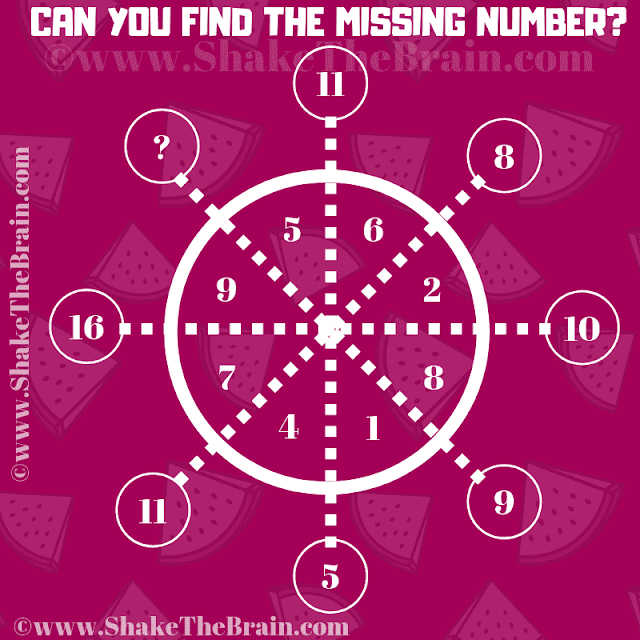Engage in an easy maths logical reasoning puzzle: a captivating number game question. Challenge your mind and find the answer.

It is an easy maths puzzle for school kids. Solving this circle puzzle will help you to test your maths and logical skills. In this circle maths puzzle, you are shown some numbers inside and outside the circle. Your challenge is to find the relationship among these given numbers in the Circle and then find the value of the missing number which will replace the question mark.Can you find the value of the missing number?

The answer to this "Easy Maths Puzzle", can be viewed by clicking on the answer button.